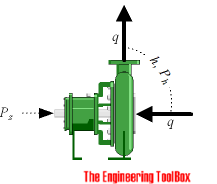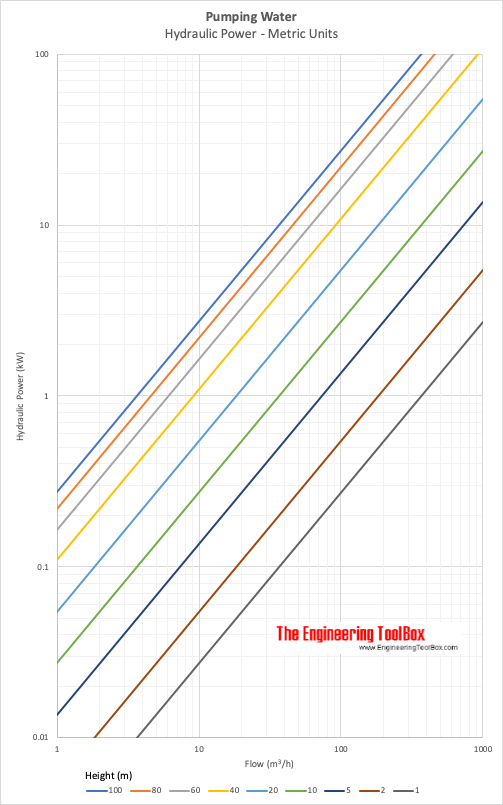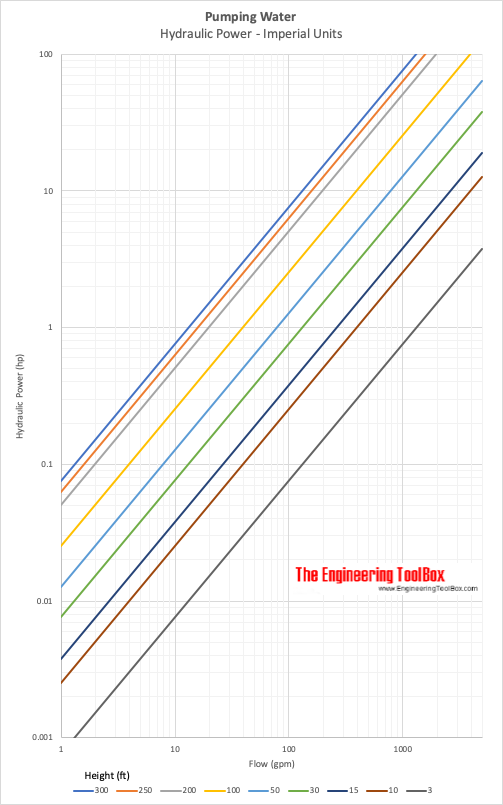Engineering ToolBox - Resources, Tools and Basic Information for Engineering and Design of Technical Applications!

# Pump Power Calculator

## Calculate pumps hydraulic and shaft power.

### Hydraulic Pump Power

The ideal hydraulic power to drive a pump depends on

• the mass flow rate the
• liquid density
• the differential height- either it is the static lift from one height to an other or the total head loss component of the system - and can be calculated like

Ph(kW) = q ρ g h / (3.6 106)

= q p / (3.6 106)                  (1)

where

Ph(kW) = hydraulic power (kW)

q = flow (m3/h)

ρ = density of fluid (kg/m3)

g = acceleration of gravity (9.81 m/s2)

p = differential pressure (N/m2, Pa)

The hydraulic Horse Power can be calculated as:

Ph(hp)Ph(kW) / 0.746                                  (2)

where

Ph(hp) = hydraulic horsepower (hp)

Or - alternatively

Ph(hp) = qgpm hft SG / (3960 η)                              (2b)

where

qgpm = flow (gpm)

SG = Specific Gravity (1 for water)

η = pump efficiency

### Example - Power pumping Water, SI Units

1 m3/h of water is pumped a head of 10 m. The theoretical pump power can be calculated as

Ph(kW) = (1 m3/h) (1000 kg/m3) (9.81 m/s2) (10 m) / (3.6 106)

= 0.027 kW

### Example - Power pumping Water, Imperial Units

600 gpm of water is pumped a head of 110 ft. The efficiency ofthe pump i s 60% (0.6) and the specific gravity of water is 1. The pump shaft power can be calculated as

Ph(kW) = (60 gpm) (110 ft) (1) / ((3960) (0.6))

= 27.8  hp

### Shaft Pump Power

The shaft power - the power required transferred from the motor to the shaft of the pump - depends on the efficiency of the pump and can be calculated as

Ps(kW) Ph(kW) / η                                     (3)

where

Ps(kW)  = shaft power (kW)

η = pump efficiency

### Online Pump Calculator - SI-units

The calculator below can used to calculate the hydraulic and shaft power of a pump:

q - flow (m3/h)

ρ - density of fluid (kg/m3)

g - gravity (m/s2)

η - pump efficiency

### Online Pump Calculator - Imperial units

The calculator below can used to calculate the hydraulic and shaft power of a pump using Imperial units:

q - flow capacity (gpm)

γ - specific weight of fluid (lb/ft3)

g - gravity (ft/s2)

η - pump efficiency

### Related Mobile Apps from The Engineering ToolBox

- free apps for offline use on mobile devices.## Related Topics

• ### Pumps

Piping systems and pumps - centrifugal pumps, displacement pumps - cavitation, viscosity, head and pressure, power consumption and more.

## Related Documents

• ### Centrifugal Pumps

An introduction to Centrifugal Pumps.
• ### Centrifugal Pumps - Shut-Off Head

Centrifugal pumps and maximum shut-off head.
• ### Centrifugal Pumps - Viscosity Influence

Hydrodynamic losses through pumps depends on fluid viscosities.
• ### Efficiency in Pumps or Fans

The overall pump and fan efficiency is the ratio power gained by the fluid to the shaft power supplied.
• ### Electrical Motors - Shaft Power vs. Voltage and Current

Calculate electric motors shaft power.
• ### Hydraulic Pump Motor Sizing

Motor size vs. flow rate, shaft torque, shaft power and hydraulic power.
• ### Positive Displacement Pumps

Introduction tutorial to positive displacement pumps basic operating principles.
• ### Power

Power is the rate at which work is done or energy converted.
• ### Power Gained by Fluid from Pump or Fan

Calculate the power gained by fluid from an operating pump or fan.
• ### Pump - Temperature Rise vs. Volume Flow

Calculate temperature rise vs. volume flow in pumps.
• ### Pumping Water - Energy Cost Calculator

Calculate the energy cost of pumping water.
• ### Pumping Water - Required Horsepower

Horsepower required to pump water.
• ### Pumps, Fans and Turbines - Horsepower

British Horse Power as used for pumps, fans and turbines - and how to convert to other units.
• ### System Curve and Pump Performance Curve

Utilize the system curve and the pump performance curve to select the proper pump for a particular application.
• ### Turbo Machines - Specific Work done by Pumps, Compressors or Fans

Calculate specific work done by pumps, fans, compressors or turbines.
• ### Work done by Force

Work done by a force acting on an object.

## Engineering ToolBox - SketchUp Extension - Online 3D modeling!

Add standard and customized parametric components - like flange beams, lumbers, piping, stairs and more - to your Sketchup model with the Engineering ToolBox - SketchUp Extension - enabled for use with older versions of the amazing SketchUp Make and the newer "up to date" SketchUp Pro . Add the Engineering ToolBox extension to your SketchUp Make/Pro from the Extension Warehouse !

We don't collect information from our users. More about

## Citation

• The Engineering ToolBox (2003). Pump Power Calculator. [online] Available at: https://www.engineeringtoolbox.com/pumps-power-d_505.html [Accessed Day Month Year].

Modify the access date according your visit.

9.19.12